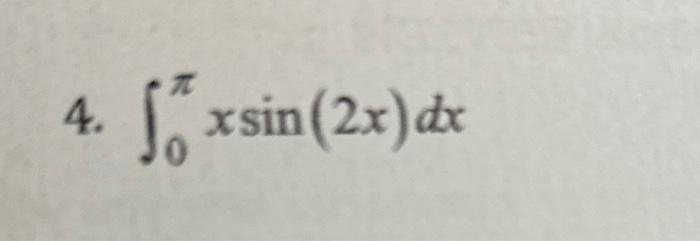Home / Expert Answers / Calculus / integrate-using-tabular-step-by-step-work-nbsp-int-0-pi-x-sin-2-x-d-x-pa432

# (Solved): integrate using tabular step by step work  $$\int_{0}^{\pi} x \sin (2 x) d x$$ ...

integrate using tabular step by step work$$\int_{0}^{\pi} x \sin (2 x) d x$$

We have an Answer from Expert